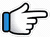# Teri Jhalak Srivalli – Keyboard Notes## Teri Jhalak Asharfi Srivalli Keyboard Notes

For Teri Jhalak Srivalli Notes as Western or Indian Notations, Click –

Western – CDE FGAB

Indian – srg mpdn

Hindi – सारेग मपधनि

## Keyboard Notes for Teri Jhalak Srivalli – Indian Notation (srg mpdn)

CDE FGAB

सारेग मपधनि

Music:
Music notes are given after the song notes.

### Part 1

ggn         nn       n    dnn       n
Nazrein   milte   hi   nazron   se

nnd         n ndD
Nazron   ko_ churaye
Or
nd            n ndD

### Video Tutorial for Teri Jhalak Asharfi Srivalli Keyboard Notes – Slow

gn       n    nn       nd
Kaisi   ye   haya   teri

n     n     nnd      n    ndD
Jo   tu   palkon   ko   jhukaye

### Part 2

d        d    dDM        DMg
Rab   jo    poshida    hai~~
Or
d       d    nDM        DMg

dd       nDM     DMg
Usko   nihare    tu~~

d~    d~    ndDM      DMg
Aur_ jo     garveeda   hai
Or
dd   d     ndDM      DMg

gM         gG     g  g*
Usko      taale   tu~~
Or
gDM    gG     g  g*

### Part 3

g*g*   g*g*g*  g*M*D*~ M*
Teri    jhalak    asharfi

D*M*   d*D*M*g*
Srival_li~~~
Or
D*M*D*M*g*
D*M*   D*d*D*  M*g*

r*r*        r*R*r*      R*r*n
Or
r*r*      r*R*r*      R*r*g*  r*R*n

g*g*   g*g*g*      g*n*n*D*~
Teri     jhalak      asharfi
Or
g*g*   g*g*g*     g*n*D*~

D*M*D*M*g*
Srivalli

r*r*          r*R*r*        R*r*g*r*R*n
Baatein   kare_ do    harfi~~

### Part 4

g*~~    g*g*     g*r*R*  r*R*n
Hmm   Saara    zama~na  ~~~
Or
g*~~    g*g*    M*g*r*R*~

nR* nD  dn
hai_ mere_ peeche

g*      g*     g*r*R*  r*R*n
Par    ye     deewana   ~~

nR* nd   Dd
hai_ tere_  peeche
Or
n     R*n  Dd

g*     g*    M*g*r*   R*   n
Sar   ye     jhukne   na   du
Or
g*     g*    g*r*R*  r*R*  n

nR*n      D    dn
duniya   ke   aage

g*     g*M*    g*r*      r*R*~
Par    teri       payal   dekhu
Or
g*     g*M*   g*r*    R*n~
g*     g*g*    r*R*   r*n~

nR*n     D~   dd~
kar ke   sar    niche
Or
nR*n     d~    Dd~

### Part 5

n      R*r*R*         nR*      r*R*
Na   tamanna     heera   panna

nR*           r*   g*
Mujhko   hai   bas

r*R*      r*R*n
tera      ban-na
Or
r*R*      r*n

n~   R*r*       R*n    R*r*         R*
Ek    jhalak   teri     aankon   mein

g*   g*  g*g*       r*M*g*~
Khwaa_b_saja    jaye
Or
g*  g*  g*g*       g*g*~

### Repeat Part 3

g*g*   g*g*g*  g*M*D*~ M*
Teri    jhalak    asharfi

D*M*   d*D*M*g*
Srival_li~~~
Or
D*M*D*M*g*
D*M*   D*d*D*  M*g*

r*r*        r*R*r*      R*r*n
Or
r*r*       r*R*r*      R*r*g*  r*R*n

g*g*  g*g*g*   g*n*n*D*~
Teri   jhalak      asharfi
Or
g*g*  g*g*g*   g*n*D*~

D*M*D*M*g*
Srivalli

r*r*          r*R*r*   R*r*g*r*R*n
Baatein   kare_ do    harfi~~

### Part 6

Wil be Updated:

g*g* g*r*R* r*R*n
nR* nD dn
g* g* g*r*R* r*R*n
nR* nd Dd

Uske muqable thodi tu bhali

g* g* M*g*r* R* n
nR*n D dn
g* g*M* g*r* r*R*~
nR*n D~ dd~

Jaise hi solwan chadh jaye savan
Tu kya har ladki dikhe phoolo ki kali

n R*r*R* nR* r*R*
nR* r* g*
r*R* r*R*n

Bans pe lipti laal saree
Wo bhi dikhe raj kumari

n~ R*r* R*n R*r* R*
g* g* g*g* r*M*g*~

Jhumke bindi aur gajre se
Roop nikhar jaye Fir bhi

### Music Notes:

Can be played in higher octave

Part 1
gDMD~   Mg  Mr
gDMD~   MD  g *n
Or
gDMD~   M g~ r
gDMD~   M  g

Part 2
gDMD~   M  g  r
gMn     dnD
Or
gDMD~   M  g  r
gDR*~     nD

Part 3
gDMD~   M  g  r
gDMD~   M  g

Part 4
gDMD~   M  g  r
gMn  R*nd  nD
Or
gDMD~   M  g  r
gDnR*~    dnD

Song List

Bhajan List

New Song List